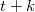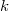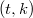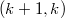﻿

### Vector space partitions and designs Part II – constructions

#### Abstract

This article is the second part and companion article to Part I on the basictheory of what are called focal-spreads; partitions of finite vector spacesof dimension$t+k$ by one subspace of dimension$t$ (the `focus') and theremaining subspaces of dimension$k$, a `focal-spread of type$(t,k)$'$.$ InPart I, additive focal-spreads are shown to be equivalent to additivepartial spreads. Focal-spreads of type$(k+1,k)$ also produce$%2-(q^{k+1},q,1)$-designs, \ and various other double and triple-spreads.Also, in Part I, there are two different methods given to constructfocal-spreads, one of which is due to Beutelspacher, the other method beinga coordinate method similar to the theory available for translation planes.Here, we shall give a new construction that we term "going up," which alsoallows a specification of certain subplanes of the focal-spread.

DOI Code: 10.1285/i15900932v30n2p101

Keywords: vector space partition; designs; focal-spread; going up

Full Text: PDF